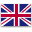Shenmao Capacitors specialized in aluminum electrolytic capacitors from 1970

ShIP toEnglish

# Switching power supply capacitor loss_power engineer needs to see

by：Shenmao     2021-06-06
Energy conversion systems must have energy consumption. Although 100% conversion efficiency cannot be obtained in practical applications, a high-quality power supply efficiency can reach a very high level, with an efficiency close to 95%. The working efficiency of most power ICs can be measured under specific working conditions, and these parameters are given in the data sheet. Generally, manufacturers will give actual measurement results. Most of the losses of the switching power supply come from switching devices (MOSFET and diode), and a small part of the losses come from inductance and capacitance. However, if very cheap inductors and capacitors (with higher resistance) are used, the loss will increase significantly. When choosing an IC, you need to consider the controller's architecture and internal components in order to obtain high-efficiency indicators. Capacitive losses are contrary to ideal capacitor models, and the actual physical properties of capacitive elements cause several losses. Capacitors mainly play a role in stabilizing and filtering input/output noise in SMPS circuits (Figure 1). These losses of capacitors reduce the efficiency of the switching power supply. These losses are mainly manifested in three aspects: equivalent series resistance loss, leakage current loss and dielectric loss. The resistive loss of the capacitor is obvious. Since current flows into and out of the capacitor during each switching cycle, the inherent resistance (RC) of the capacitor will cause a certain amount of power dissipation. Leakage current loss is the power loss due to the resistance (RL) of the capacitor's insulating material causing a small current to flow through the capacitor. Dielectric loss is more complicated. Because of the AC voltage applied across the capacitor, the electric field of the capacitor changes, which polarizes the dielectric molecules and causes power loss. All three losses are reflected in the typical loss model of the capacitor (left part of the figure), and each loss is represented by a resistance. The power of each loss related to capacitive energy storage is expressed by the power dissipation factor (DF), or the loss tangent (δ). The DF of each loss can be obtained by the ratio of the real part to the imaginary part of the capacitor impedance, and each loss can be inserted into the model separately. In order to simplify the loss model, the contact resistance loss, leakage current loss and dielectric loss concentration in the figure are an equivalent series resistance (ESR). ESR is defined as the part of the capacitive impedance that consumes active power. When calculating the capacitance impedance model and calculating ESR (the real part of the result), ESR is a function of frequency. This correlation can be proved in the following simplified ESR equation: where DFR, DFL, and DFD are the power dissipation coefficients for contact resistance, leakage current, and dielectric loss. Using this equation, we can observe that as the signal frequency increases, both leakage current loss and dielectric loss decrease until the contact resistance loss begins to dominate from a higher frequency point. Above this frequency point (this parameter is not included in the formula), ESR tends to increase due to the skin effect of high-frequency alternating current. Many capacitor manufacturers provide ESR graphs showing the relationship between ESR and frequency. For example, TDK provides ESR curves for most of its capacitor products. Refer to these graphs corresponding to the switching frequency to obtain the ESR value. However, if the ESR graph is not available, the ESR can be roughly estimated from the DF specification in the capacitor data sheet. DF is the overall DF of the capacitor (including all losses). ESR can also be estimated according to the following formula: No matter which method is used to obtain the ESR value, intuition tells us that high ESR will reduce the efficiency of the switching power supply. Since the input and output capacitors are in each The switching cycle is charged and discharged through ESR. This results in I2×RESR power loss. This loss (PCAP(ESR)) can be calculated according to the following formula: PCAP(ESR)u003d ICAP(RMS)2×RESR In the formula, ICAP(RMS) is the RMS of the AC current flowing through the capacitor. For the output capacitor of the step-down circuit, the effective value RMS of the inductor ripple current can be used. The calculation of the RMS current of the input filter capacitor is more complicated. A reasonable estimate can be obtained according to the following formula: ICIN(RMS)u003d IOUT/VIN× [VOUT(VIN- VOUT)]1/2 Obviously, in order to reduce the power loss of the capacitor , Low-ESR capacitors should be selected to help the SMPS power supply reduce ripple current. ESR is the main cause of output voltage ripple, so choosing a low-ESR capacitor not only improves efficiency, but also gains other benefits. Generally speaking, capacitors of different types of dielectrics have different ESR levels. For a specific capacity and rated voltage, aluminum electrolytic capacitors and tantalum capacitors have higher ESR values u200bu200bthan ceramic capacitors. Polyester and polypropylene capacitors have ESR values u200bu200bbetween them, but these capacitors are larger in size and are rarely used in SMPS. For a given type of capacitor, a larger capacity and lower fS can provide a lower ESR. Large-size capacitors usually also reduce ESR, but electrolytic capacitors will bring a larger equivalent series inductance. Ceramic capacitors are considered a good compromise choice. In addition, under the condition of a certain capacitance value, a lower capacitor rated voltage also helps to reduce ESR.
Shenzhen Shen MaoXin Electronics Co., Ltd. continued to crave a more intense, personalized workout experience.
Shenmao Capacitors has a whole range of different items to help you make an informed choice every time you make a purchase. Check it!
electrolytic capacitor is produced by Shenzhen Shen MaoXin Electronics Co., Ltd.’s professional skills in high technology.
Shenzhen Shen MaoXin Electronics Co., Ltd.’s mission is to provide you with an outstanding member/Customer benefit that helps you meet your organization’s objectives.
Custom message
Chat Online 编辑模式下无法使用